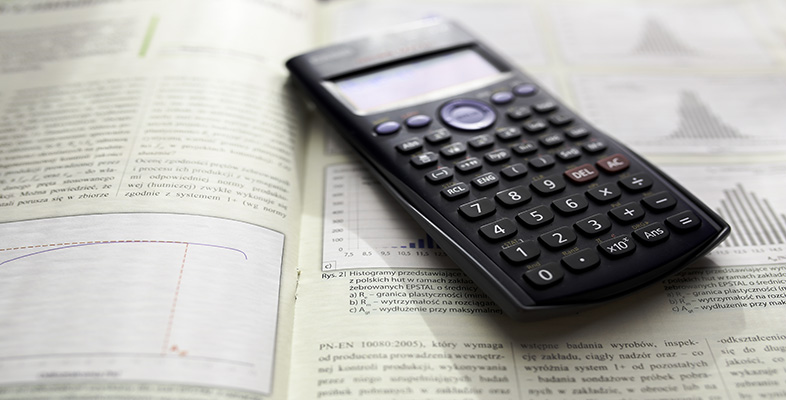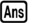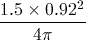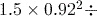Science, Maths & Technology

### Become an OU studentUsing a scientific calculator

Start this free course now. Just create an account and sign in. Enrol and complete the course for a free statement of participation or digital badge if available.

# 4.1 Reusing a previous result

An alternative approach to our calculation is to calculate the denominator of the fraction first, and then divide the numerator by this.

You could write down the answer to the first part of the calculation on paper, and enter it into the calculator again. However, it is possible that you may make an error either in writing down the number or in typing it into the calculator. A better method is to use the fact that the calculator retains the last calculated answer, which can then be inserted in the subsequent calculation using thekey located at the bottom of the keypad.

Note that thekey only remembers the result of your last calculation.

## Activity 11 Bottom first!

Use your calculator to calculate the value of the denominator of, then complete the calculation by finding the value ofto 3 significant figures.